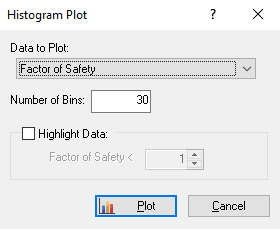The Rocscience International Conference 2021 Proceedings are now available. Read Now

# Histogram Plot

To plot a histogram of results after a Probabilistic analysis:

1. Select Plot Histogramon the toolbar or the Statistics menu to open the Histogram Plot dialog.2. Select the data you want to plot in the Data to Plot (e.g., Factor of Safety or one of the parameters you've defined as a random variable).
3. Enter a Number of Bins. The default is 30, but any value between 2 and 100 can be entered.
4. To highlight data in the plot with a Factor of Safety lower than a certain number, select the Highlight Data check box and enter a value ( the default = 1).
5. Click Plot.
The histogram is generated in the Histogram View, and the mean, standard deviation, and minimum and maximum values for the data plotted are displayed at the bottom, as shown in the figure below.## Best Fit Distribution

If the Data to Plot is Factor of Safety, a Best Fit Distribution is determined and can be displayed on the histogram along with the distribution parameters.

## Input Distribution

If the Data to Plot is a random variable, the Input Distribution can be plotted.

## Right Click Options

A number of plotting and export options are available in the Histogram View right-click menu. These include:

• Vertex Markers
• Relative Frequency
• Input Distribution
• Best Fit Distribution
• Chart Properties
• Change Plot Data
• Copy
• Copy Data
• Plot in Excel
• Export Image